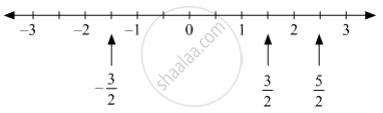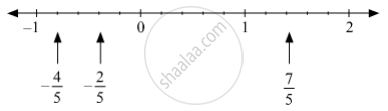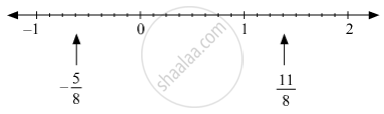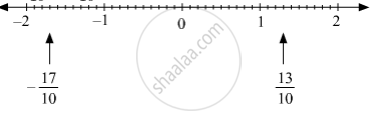# Show the Following Numbers on a Number Line. Draw a Separate Number Line for Each Example. - Mathematics

Sum

Show the following numbers on a number line. Draw a separate number line for each example.

(1)3/2 , 5/2 , -3/2

(2)7/5 , (-2)/5 , (-4)/5

(3) (-5)/8 , 11/8

(4)13/10 , (-17)/10

#### Solution

(1)3/2 , 5/2 , -3/2 can be represented on the number line as follows.(2)7/5 , -2/5 , -4/5 can be represented on the number line as follows.(3)-5/8 , 11/8 can be represented on the number line as follows.(4) 13/10 , -17/10 can be represented on the number line as follows.Concept: Rational Numbers on a Number Line
Is there an error in this question or solution?